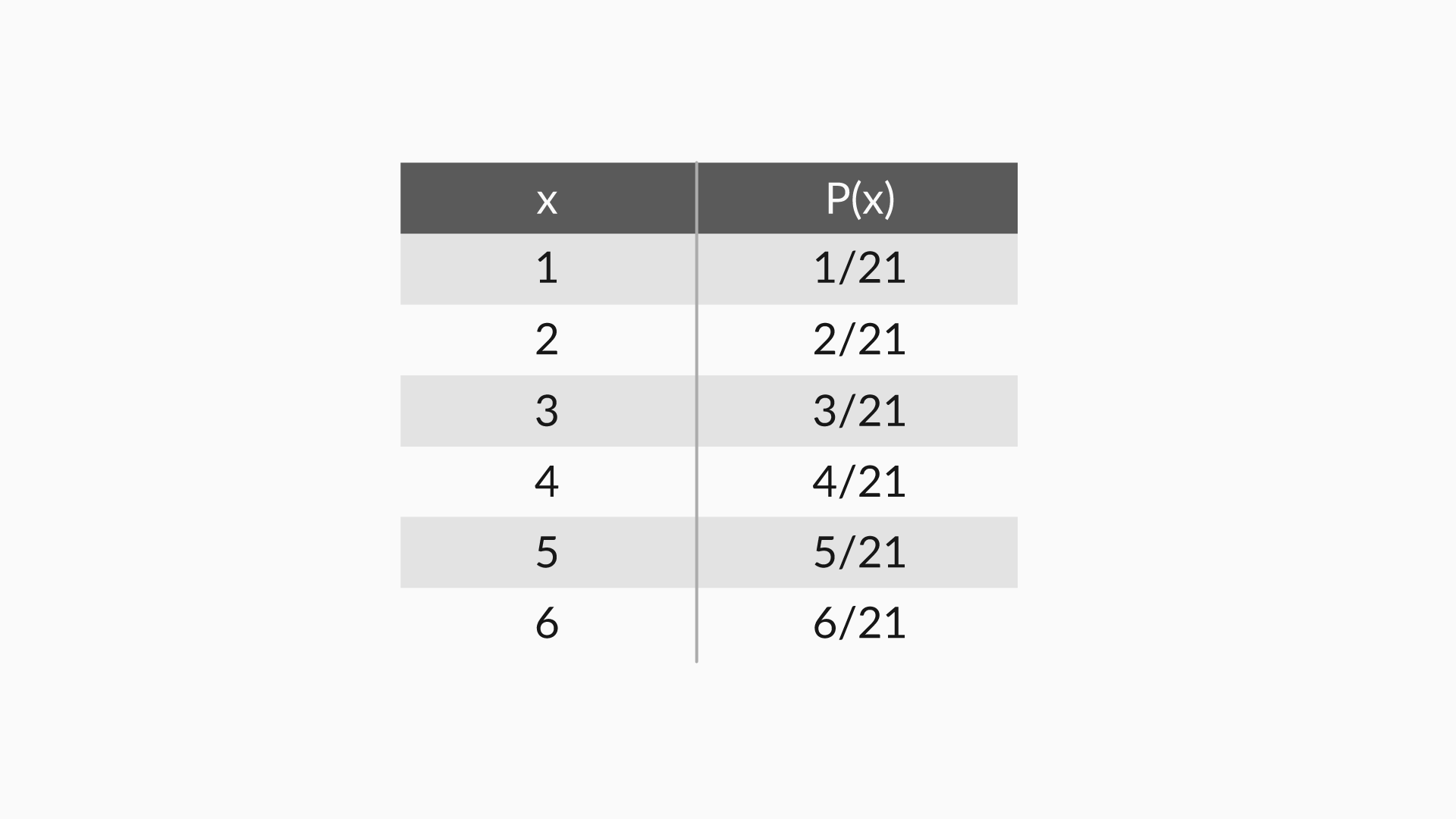# Inferential Statistics – Part 1## Introduction: Inferential Statistics :

The process of “inferring” insights from sample data is called “Inferential Statistics”

### Basics of Probability:

In the topic we will go through:

• Basic definition of probability
• Multiplication rule of probability